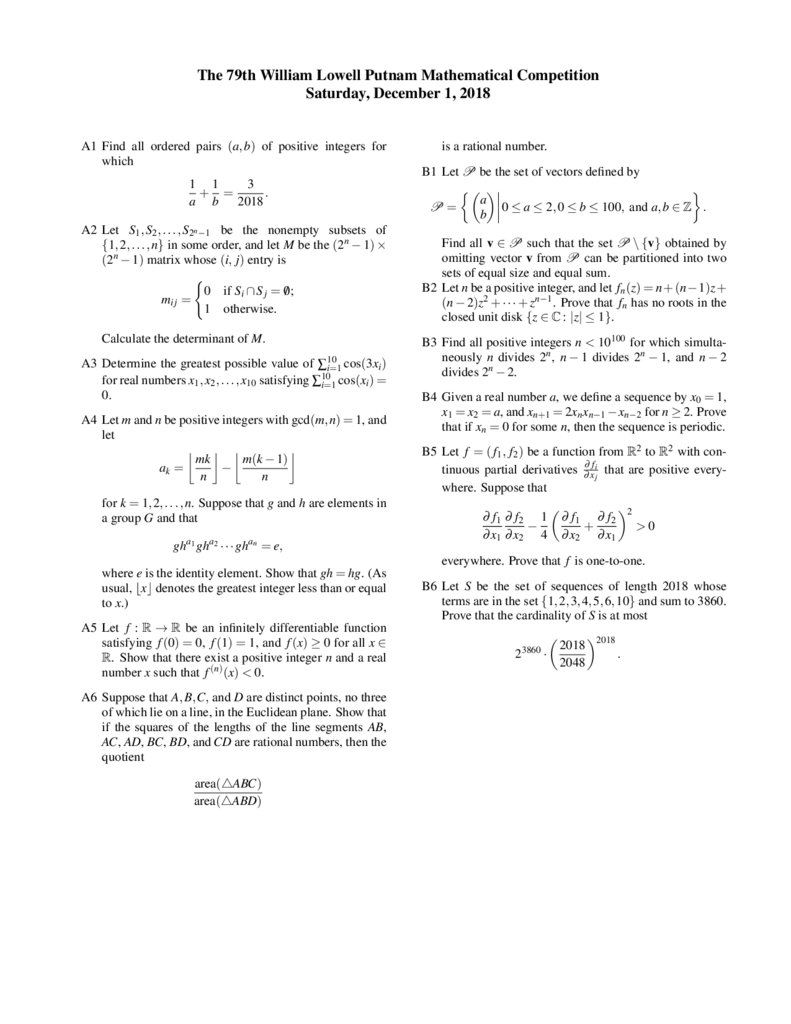# The 79th William Lowell Putnam Mathematical

## 1.

The 79th William Lowell Putnam Mathematical Competition
Saturday, December 1, 2018
A1 Find all ordered pairs (a, b) of positive integers for
which
1 1
3
+ =
.
a b 2018
A2 Let S1 , S2 , . . . , S2n −1 be the nonempty subsets of
{1, 2, . . . , n} in some order, and let M be the (2n − 1) ×
(2n − 1) matrix whose (i, j) entry is
(
0 if Si ∩ S j = 0;
/
mi j =
1 otherwise.
Calculate the determinant of M.
A3 Determine the greatest possible value of ∑10
i=1 cos(3xi )
for real numbers x1 , x2 , . . . , x10 satisfying ∑10
i=1 cos(xi ) =
0.
A4 Let m and n be positive integers with gcd(m, n) = 1, and
let
m(k − 1)
mk

ak =
n
n
for k = 1, 2, . . . , n. Suppose that g and h are elements in
a group G and that
a1
a2
an
gh gh · · · gh = e,
is a rational number.
B1 Let P be the set of vectors defined by
a
0 ≤ a ≤ 2, 0 ≤ b ≤ 100, and a, b ∈ Z .
P=
b
Find all v ∈ P such that the set P \ {v} obtained by
omitting vector v from P can be partitioned into two
sets of equal size and equal sum.
B2 Let n be a positive integer, and let fn (z) = n+(n−1)z+
(n − 2)z2 + · · · + zn−1 . Prove that fn has no roots in the
closed unit disk {z ∈ C : |z| ≤ 1}.
B3 Find all positive integers n < 10100 for which simultaneously n divides 2n , n − 1 divides 2n − 1, and n − 2
divides 2n − 2.
B4 Given a real number a, we define a sequence by x0 = 1,
x1 = x2 = a, and xn+1 = 2xn xn−1 −xn−2 for n ≥ 2. Prove
that if xn = 0 for some n, then the sequence is periodic.
B5 Let f = ( f1 , f2 ) be a function from R2 to R2 with continuous partial derivatives ∂∂ xfij that are positive everywhere. Suppose that
∂ f1 ∂ f2 1

∂ x1 ∂ x2 4
∂ f1 ∂ f2
+
∂ x2 ∂ x1
2
>0
everywhere. Prove that f is one-to-one.
where e is the identity element. Show that gh = hg. (As
usual, bxc denotes the greatest integer less than or equal
to x.)
A5 Let f : R → R be an infinitely differentiable function
satisfying f (0) = 0, f (1) = 1, and f (x) ≥ 0 for all x ∈
R. Show that there exist a positive integer n and a real
number x such that f (n) (x) < 0.
A6 Suppose that A, B,C, and D are distinct points, no three
of which lie on a line, in the Euclidean plane. Show that
if the squares of the lengths of the line segments AB,
AC, AD, BC, BD, and CD are rational numbers, then the
quotient
area(4ABC)
area(4ABD)
B6 Let S be the set of sequences of length 2018 whose
terms are in the set {1, 2, 3, 4, 5, 6, 10} and sum to 3860.
Prove that the cardinality of S is at most
2
3860
2018
·
2048
2018
.